### Math Warm-Ups(From Steve Wyborney)Clothesline Math (Estimation 180)# Desmos:  Fractions, Decimals, and Percents on the Number Line# Math Problem of the Day

Number and Operations: Multiplication and Division

Common Core Standards

Arithmetic (PhET)

Multiplication Table Exploration

Area Model Introduction (PhET)

Missing Factors

Factor BingoNumber and Operations: Fractions

Common Core StandardsEquivalent Fractions

Sp Equivalent Fractions

Fractions on the Number Line, Equivalent Fractions, Partitioning Rectangles

Fraction Concentration

Match fractions to an area or group model.

The Fraction Game

Decomposing fractions, equivalent fractions, combining fractions.

Cover Up Fraction Game

Cùbrelo (Spanish)

Explore the relative size of fraction pieces and relationships between them.

Capture Fractions

Uncover Fraction Game

Descubrelo/Destapalo (Spanish)

Get really good at strategically exchanging fractions for equivalent fractions.

Greatest Fraction Wins!

La Fracción más Grande Gana! (Spanish)

Roll and place each digit as you explore the role of the numerator & denominator  in the value of a fraction as well as model and compare fractions. For 4th and 5th grades, play the Greatest Sum or Greatest Difference wins!

Fraction Capture

Captura la Fracción (Spanish)

This game is like the card game “War.”  Compare fractions, justify your thinking, win cards!

Fraction War (from Fractiontalks.com)

Fraction War Video

Fraction War Cards

Fractions In-Between Game

Fracciones en el Medio (Spanish)

Compare fractions, order fractions, justify your thinking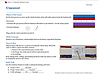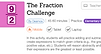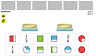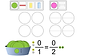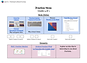Number and Operations in Base Ten: Decimals

Common Core StandardsDesmos:  The Decimal Challenge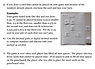Target 1

En el Blanco 1 (Spanish)

Players choose cards to make 2 decimal numbers that have a sum as close to 1 as possible.

Target 2

En el Blanco 2 (Spanish)

The Place Value Game

Juego de Valor Posicional (Spanish)

Place Value Game Demo Video

Virtual Spinner

Decimal Place Value

Comparar con Decimales (Spanish)

Digital Tool:  Modeling Decimals

Compare decimals - like the card game War.

Decimal Double Compare

Comparación Decimal Doble (Spanish)

Digital Tool:  Modeling Decimals

Smaller to Larger - Decimals

Decimales de Menor a Mayor (Spanish)

Digital Tool:  Modeling Decimals